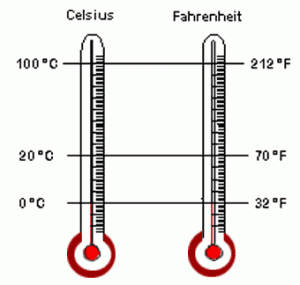# Fahrenheit to Celsius Conversion

You use Fahrenheit when you’ve got somethingcooking in the oven. However, hospitals may indicate your temperature in degrees Celsius. There are times when you need one or the other of these two temperature scales. When a child is sick, a parent would usually use degree Fahrenheit to indicate how high their child’s body temperature is. Converting from Fahrenheit to Celsius and vice versa is a fact of life and a skill that anyone should have.

### Fahrenheit to Celsius Conversion Options

There are several options open to you if you want to convert temperatures from Fahrenheit to Celsius. If you have a way to access the Internet then all you need to do is open a web browser and go to any temperature conversion sites online. Once you’re on one all you have to do is select the what temperature scale you’re using, enter the temperature you need to convert, indicate what temperature scale you want to convert to and then hit enter or click ‘OK’.

Some of these sites will just have you enter the amount and the temperature scales you want to use. At any rate, the on screen options you will find in the many temperature conversion sites are pretty to understand. However, if you don’t have ready access to the Internet you will have to calculate Fahrenheit to Celsius on your own. Given this situation, it helps to have a calculator ready to deal with the math. In case you don’t have a calculator on you then you’ll just have to do the calculations on paper, unless of course you can do it in your head.

### Conversion Formulas

To convert Fahrenheit to Celsius, you should know the formula by which to switch from one temperature scale to the other. The formula to convert Celsius to Fahrenheit is: (degrees Celsius x 9/5) + 32. The formula for Fahrenheit to Celsius on the other hand is: (degrees Fahrenheit – 32) x 5/9.

Translating the crazy formula into lay men’s terms, if you want to convert Celsius to Fahrenheit multiply your temperature in Celsius by nine. Next, divide the result by five. And finally, add 32 degrees and you have your temperature in Fahrenheit. To convert from Fahrenheit to its equivalent in degrees Celsius deduct 32 degrees from what you have in Fahrenheit, multiply the result by five, and finally divide by nine.

Take note that the result you get in Celsius should be smaller than what you have in Fahrenheit, not the other way around. Remember that when you use either of these two scales that you are only dealing with the same thing – temperature.